﻿ 基于改进多层编码遗传算法的多配送中心车辆路径优化方法研究 Research on Multi-Distribution Center Vehicle Routing Optimization Method Based on Improved Multi-Layer Coding Genetic Algorithm

Open Journal of Transportation Technologies
Vol. 08  No. 03 ( 2019 ), Article ID: 30236 , 11 pages
10.12677/OJTT.2019.83027

Research on Multi-Distribution Center Vehicle Routing Optimization Method Based on Improved Multi-Layer Coding Genetic Algorithm

Lunhui Xu1, Yuchao Cao2, Baoshan Huang1

1School of Industrial Automation, Zhuhai University, Beijing Institute of Technology, Zhuhai Guangdong

2School of Civil Engineering and Transportation, South China University of Technology, Guangzhou Guangdong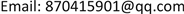Received: Apr. 29th, 2019; accepted: May 9th, 2019; published: May 16th, 2019ABSTRACT

In order to improve logistics transportation efficiency and reduce unnecessary resource consumption, comprehensive planning of a most efficient vehicle distribution route has become a hot issue in the next logistics transportation research. There are still few studies on the situation of multiple distribution centers. The Vehicle Routing Optimization Problem (VRP) is derived from the Traveling Salesman Problem (TSP), which we classify as a non-deterministic polynomial (NP) complete combinatorial optimization problem. In the context of traffic logistics vehicle routing, this paper firstly constructs a mathematical model from the conceptual analysis of VRP problem, and then describes the theoretical basis and strategic thinking of the core algorithm—genetic algorithm to solve this problem. Finally, by adding multi-factor analysis, the comprehensive cost of evaluation is improved, and the improved multi-encoding genetic algorithm is applied to solve the multi-distribution center VRP problem, and the improvement is explored in the process.

Keywords:VRP Problem, Genetic Algorithm, Multi-Layer Coding, Transportation, Logistics, Dispatching, Path Optimization

1北京理工大学珠海学院工业自动化学院，广东 珠海

2华南理工大学土木与交通学院，广东 广州1. 引言

1.1. 选题背景

1.2. 国内外研究现状

2. 车辆调度优化问题分析

2.1. 多配送中心车辆调度问题的数学模型

①每条配送路径上各需求商的需求量之和不超过车辆的载重量；

②每条配送路径的长度不超过车辆一次配送的最大行驶距离；

③每个需求商的需求必须满足，且只能由一台车辆送货。

$\mathrm{min}Z={\sum }_{h=1}^{H}\left\{{\sum }_{k=1}^{{K}_{h}}\left[{\sum }_{i=1}^{{n}_{hk}}{d}_{{r}_{hk\left(i-1\right)}{r}_{hki}}+{d}_{{r}_{hk{n}_{hk}}{r}_{hk0}}\ast sign\left({n}_{hk}\right)\right]\right\}$ (1)

$\text{s}.\text{t}.\text{\hspace{0.17em}}\text{\hspace{0.17em}}\text{\hspace{0.17em}}{\sum }_{i=1}^{{n}_{hi}}{q}_{h{r}_{hki}}\le {Q}_{hk}$ (2)

${d}_{{r}_{hk\left(i-1\right)}{r}_{hki}}+{d}_{{r}_{hk{n}_{hk}}{r}_{hk0}}\ast sign\left({n}_{hk}\right)\le {D}_{hk}$ (3)

$0\le {n}_{k}\le {L}_{h}$ (4)

${\sum }_{k=1}^{{K}_{h}}{n}_{hk}={L}_{h}$ (5)

${\sum }_{h=1}^{H}{L}_{h}=M$ (6)

${R}_{nk}=\left\{{r}_{nki}|{r}_{nki}\in \left\{1,2,\cdots ,{L}_{h}\right\},i=1,2,\cdots ,{n}_{hk}\right\}$ (7)

${R}_{hk1}\cap {R}_{hk2}=\varnothing ,\text{\hspace{0.17em}}\forall h{k}_{1}\ne h{k}_{2}$ (8)

$sign\left({n}_{hk}\right)=\left\{\begin{array}{l}1\text{\hspace{0.17em}}\text{\hspace{0.17em}}\text{\hspace{0.17em}}{n}_{hk}\ge 1\\ 0\text{\hspace{0.17em}}\text{\hspace{0.17em}}\text{\hspace{0.17em}}\text{\hspace{0.17em}}其他\end{array}$ (9)

2.2. 遗传算法

3. 车辆调度优化问题的建模

3.1. 遗传算法解决问题的基本思想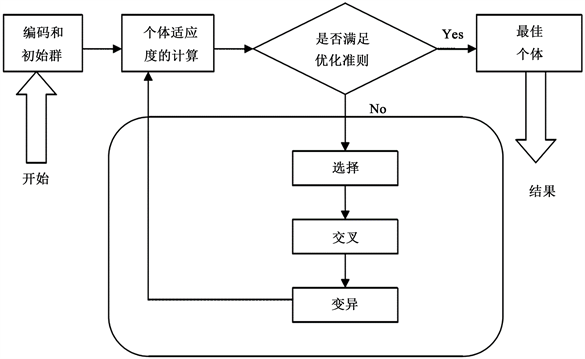Figure 1. SGA flow diagram

3.2. VRP问题的影响因素分析

1) 运输距离；2) 运输环节；3) 运输相关的运营成本；4) 货物数量

1) 与时间相关的因素：a) 货物的运到期限，b) 货物的在途时间；2) 与交货质量相关的因素；3) 线路状态；4) 信息化管理水平。

3.3. 影响因素的确定以及评价标准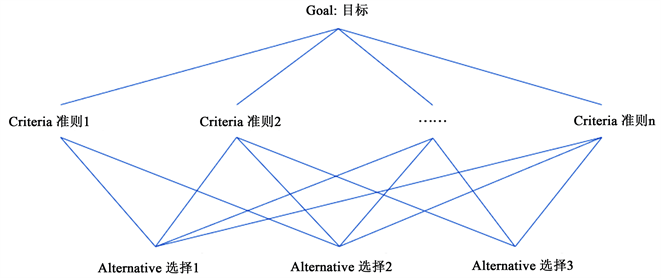Figure 2. Hierarchical analysis flow diagram

1) 建立递阶层次结构模型；

2) 构造出各层次中的所有判断矩阵；

3) 层次单排序及一致性检验；

4) 层次总排序及一致性检验。Table 1. Analytic hierarchy importance reference table

weight vector: 0.37721 0.1619 0.28694 0.082043 0.024833 0.025908 0.015824 0.025346

CI = 按照各比例重要度，以及影响水平，本文选取运输距离，货物数量(重量)，货物的在途时间，线路状态(对道路的破坏水平)四个指标进行影响因子的构建：

${c}_{ij}=\alpha \cdot s+\beta \cdot m+\gamma \cdot t+\delta \cdot d\text{\hspace{0.17em}}\text{\hspace{0.17em}}\text{\hspace{0.17em}}其中\left(\alpha +\beta +\gamma +\delta =1\right)$ (10)

4. 多层编码遗传算法解决VRP问题的实现

4.1. 模型构建

1) 供应集 $M=\left\{{m}_{1},{m}_{2},\cdots ,{m}_{m}\right\}$ ，mj表示第j个需求点， $j=1,2,\cdots ,m$

2) 需求集 $P=\left\{{p}_{1},{p}_{2},\cdots ,{p}_{n}\right\}$ ，mj表示第i个供应点， $i=1,2,\cdots ,n$

3) 路径序列集 $OP=\left\{o{p}_{1},o{p}_{2},\cdots ,o{p}_{n}\right\}$$o{p}_{i}=\left\{o{p}_{i1},o{p}_{i2},\cdots ,o{p}_{ik}\right\}$ ，表示供应商车辆所运送对应物资opi的运输批次。

4) 可提供相关所需物资的供应商的集合表示为

$OPM=\left\{o{p}_{i1},o{p}_{i2},\cdots ,o{p}_{ik}\right\}$

5) 时间矩阵T， ${t}_{ij}\in T$ ，表示第i个供应商pi第j批次到达目的地的时间。

6) 代价矩阵C， ${c}_{ij}\in C$ ，表示第i个供应商pi第j批次到到达目的地的各方面代价总和。

4.2. 算法实现

1) 个体编码

[2 4 3 1 1 2 3 4 2 1 3 3 2 2 1 3]

2) 适应度值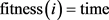(11)

3) 选择操作

$pi\left(i\right)=\text{Fitness}\left(i\right)/\underset{i-1}{\overset{n}{\sum }}\text{Fitness}\left(i\right)$(12)

4) 交叉操作

5) 变异操作

6) 计算交叉以及编译操作后适应度(即时间代价)值。

4.3. 算例

4.3.1. 配送案例Table 3. Supply and demand materials supply and demand correspondence table

${C}_{ij}=30×0.4154+10×0.1703+3×0.3159+1×0.098$

4.3.2. 配送方案验证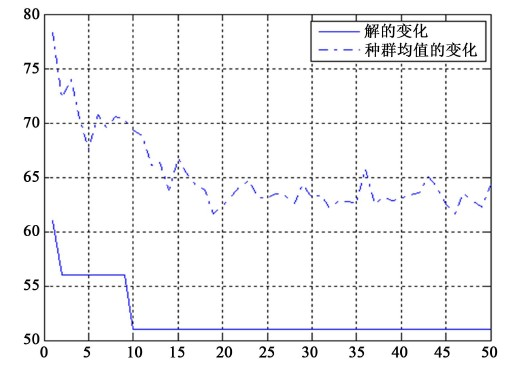Figure 3. Iteration number map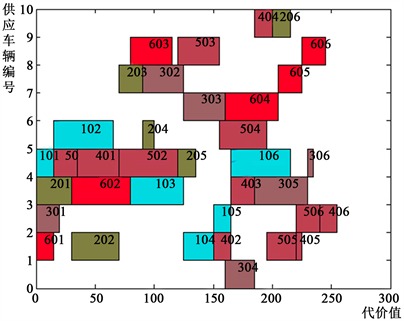Figure 4. Vehicle dispatch map

5. 结论

1) 对于物流运输中多配送中心，构建了相应数学模型进行描述。

2) 应用改进的多层编码遗传算法加入多影响因子构建了一个解决多配送中心的车辆调度及路径规划问题的方法。

3) 通过实际案例需求，构建了可视化表格进行问题描述，应用所研究的方法，证实可以得到一个完整的全局代价最小的路径规划网络。

Research on Multi-Distribution Center Vehicle Routing Optimization Method Based on Improved Multi-Layer Coding Genetic Algorithm[J]. 交通技术, 2019, 08(03): 222-232. https://doi.org/10.12677/OJTT.2019.83027

1. 1. 薛戈丽, 王建平. 一种基于蚁群算法的物流配送VRP解决方案[J]. 计算机系统应用, 2012, 21(2): 200-203.

2. 2. 崔雪丽, 马良, 范炳全. 车辆路径问题(VRP)的蚂蚁搜索算法[J]. 系统工程学报, 2004, 19(4): 418-422.

3. 3. 李向阳. 遗传算法求解VRP问题[J]. 计算机工程与设计, 2004, 25(2): 271-273+276.

4. 4. 方金城, 张岐山. 物流配送车辆路径问题(VRP)算法综述[J]. 沈阳工程学院学报(自然科学版), 2006, 2(4): 357-360.

5. 5. 钟石泉. 物流配送车辆路径优化方法研究[D]: [博士学位论文]. 天津: 天津大学, 2007.

6. 6. Wazirali, R.A., Alzughaibi, A.D. and Chaczko, Z. (2014) Adaptation of Evolutionary Algorithms for Decision Making on Building Construction Engineering (TSP Problem). International Journal of Electronics and Telecommunications, 60, 113-116. https://doi.org/10.2478/eletel-2014-0015

7. 7. Ma, H., Yang, Z., You, P. and Fei, M. (2017) Multi-Objective Biogeography-Based Optimization for Dynamic Economic Emission Load Dispatch Considering Plug-in Electric Vehicles Charging. Energy, 135, 101-111. https://doi.org/10.1016/j.energy.2017.06.102

8. 8. 贾楠, 吕永波, 付蓬勃, 等. 物流配送问题中VRP的数学模型及其求解算法[J]. 物流技术, 2007, 26(4): 54-56.

9. 9. 彭其华. 一种车辆路径优化调度算法的研究与仿真[J]. 计算机仿真, 2014, 31(5): 143-146.

10. 10. 周生伟, 蒋同海, 张荣辉. 改进遗传算法求解VRP问题[J]. 计算机仿真,2013, 30(12): 140-143+157.

11. 11. 宗辰光, 于培培, 费利鹏, 等. 基于多层编码粒子群——遗传算法融合的AGV调度问题研究[J]. 机电工程技术, 2016, 45(4): 11-14.

12. 12. 胡飞虎, 马贝龙, 杨丽, 等. 基于改进遗传算法的应急物资配送车辆调度优化问题研究[J]. 计算机应用研究, 2014, 31(10): 2928-2932+2936.

13. 13. 石彪, 池宏, 祁明亮, 等. 应急物资运输的两阶段车辆调度模型[J]. 系统工程, 2012, 30(7): 105-111.

14. 14. 饶卫振. 大规模动态车辆路径问题优化方法研究[M]. 北京: 经济科学出版社, 2018.

15. 15. 孔祥丽. 多影响因素下的导航路网数据路径规划研究[D]: [硕士学位论文]. 武汉: 武汉大学, 2018.

16. 16. 郎茂祥. 多配送中心车辆调度问题的模型与算法研究[J]. 交通运输系统工程与信息, 2006, 6(5): 65-69.

17. 17. 王保中, 康立山, 何巍. 基于实数编码遗传算法的多层神经网络BP算法[J]. 武汉大学学报(自然科学版), 1998, 44(3): 26-28.

18. 18. 谢思聪, 陈小波. 基于多层编码遗传算法的两阶段装配式建筑预制构件生产调度优化[J]. 工程管理学报, 2018, 32(1): 18-22.

19. 19. 王琛, 游伟, 袁泉, 等. 一种基于多层编码遗传算法的虚拟网络功能调度方法[J]. 信息工程大学学报, 2018, 19(3): 275-281.